2023 August 21
math

Growing up we learn about numbers and their properties by example: first we encounter numbers in counting, then how to add and subtract, and successively learn formal properties like associativity and the distributive law. People without a background in mathematics can be left with this idea that there is a formal mathematical notion of a “number”, but not have a clear formalization in mind, leading to perennial arguments like whether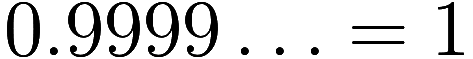.

Mathematicians, however, have not one concept of number, but many, varying from the integers to polynomial rings or function spaces or other much more exotic and specialized realms. We say things like “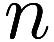bymatrices with integer elements form a ring”, meaning that addition and multiplication of such matrices satisfies certain familiar properties. Does that mean matrices are “numbers”? Well, “number” doesn’t have a formal definition in mathematics, but the word is still quite useful, so I will propose a simple informal definition: A number is a thing that can be added or multiplied.

Which is to say that being a number is roughly synonymous with being an element of a ring. What counts as “addition” and “multiplication” is left undefined; there are many binary operations in mathematics, but some “feel” more or less like addition and multiplication and the notation canonically used to describe them is often in accordance. Nothing stops you from defining your own ring operations such that elephants plus giraffes are chickens and declaring of the whole animal kingdom “Behold, a number!”, but you will be hard-pressed to get other mathematicians to use your operations or notation.

So, let us venture forth and explore the properties of one of these number systems, the field of real numbers. Often first encountered in high school, they can feel mysterious and alien to the non-mathematician, yet in reality they are very close at hand. Hopefully by the end we can bring the reals down to terra firma, as they say.

## Drawing the reals

One of the earliest questions one has when trying to understand real numbers is how to visualize them, often phrased as “how do I draw a real number in the plane?”. This can be combined with the common mistake that a real number is simply half of an ordinary number, yielding a diagram like this:

Most people realize that a real number is not found by literally dividing a number by two, but remain mystified by in what sense a real is “half” a number or how one can draw half of a number in the plane.

It need not be so complicated. Consider again our number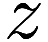, and now draw its projections down and to the left:

I have labelled these projections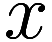and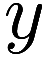. We have a simple equation relating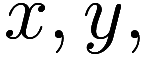and: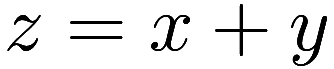Now of course there are many values ofandwhich satisfy this equation, but there is only one in which they lie on the standard axes. It is in this way that for each numberwe uniquely identify a corresponding real numberwhich we call the “real part” of. The numberis then the “other half” of, called the “imaginary part”; these imaginary parts also act like the real numbers but with a twist, so to say.

At the most basic level, that is it: a real number is simply any number that when plotted in the plane lies on the standard horizontal axis.1We leave to the reader of the slightly tricky question of what happens if you are using non-standard axes. In fact, the failure of this construction to work with non-standard axes is much of why standard axes are almost always preferred. But of course the field of real numbers would not be so useful to mathematicians (and such a subject of fascination to all math enthusiasts) were there not much more to say.

## Basic properties

Let us start with the basics. Certainly, the sum of any two real numbers if also a real number. But, thinking about the geometric interpretation of multiplication, one also notices that the product of any two reals is also real! This is like the situation with integers, which are closed under addition and multiplication. In fact, all integers are real.

The first divergence from the integers is that reals are also closed under division: the reciprocal of any nonzero real is also real. This makes the reals not just a ring but a field, a topic we will return to later.

We can recover our usual notion of addition and multiplication from addition and multiplication of reals. Suppose we have two numbers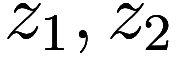, and we write them as their real and imaginary parts, making four “half” numbers: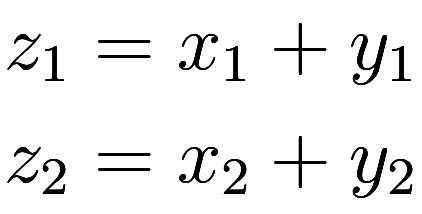Then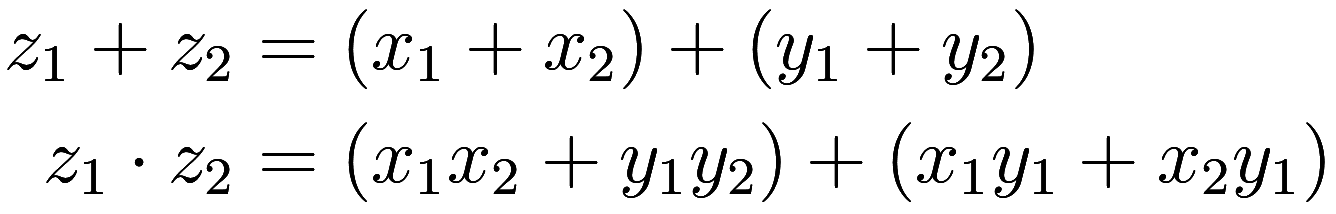With some thought one can see that a real times imaginary is imaginary, and an imaginary times imaginary is real, so expanding in this way we again have the results split into real and imaginary parts. However it can be cumbersome to keep track of all these different kinds of multiplication, and there is an immensely valuable shortcut to simplifying it. First, observe that any imaginary number can be expressed as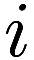times a real. Then, let us write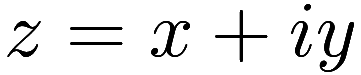where nowandare both real. This, then, is what meant by saying that a number is made up of two real numbers. I invite the reader to rework the expressions for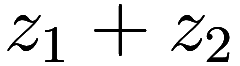and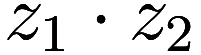with this improved definition (there is a small surprise lurking in the latter!).

## Trigonometry

Consider the familiar exponential function. Remarkably, when applied to an imaginary number, it is periodic (with period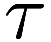)! Moreover, when we split the result into parts, we recover another famously confusing duo, sine and cosine: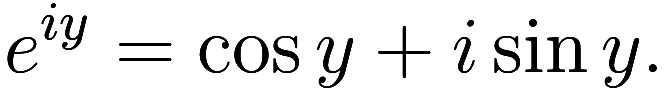Hereis real. This equation can be interpretted as describing how to convert from polar to rectangular coordinates2with the logarithm function converting the other way, if we imagine thatis in some sense an “angle” (it can be very weird at first to think of numbers as being angles).

Moreover, we can use the derivative of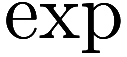to find the derivative of the trigonometric functions3using uniqueness of the decomposition, and that differentiation distributes over addition: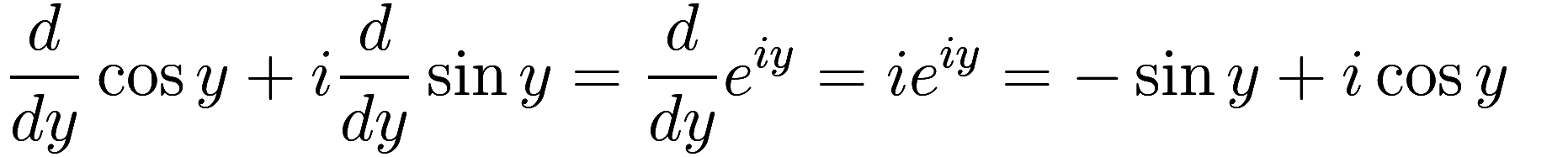and also the pythagorean formula for distance gives us the pythagorean theorem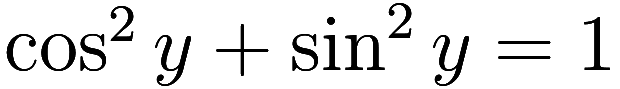as used by trigonometers.

Finally, if you did the exercise at the end of the last section, you will see that the seemingly arcane formulas for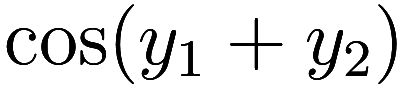and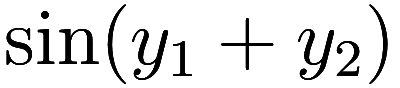are simply encoding how to multiply numbers, expressed as real parts.

## Analysis

So far we have only seen familiar concepts in unfamiliar clothing. It is when we venture into calculus (or “analysis”, when treated more rigorously) that truly new and wild phenomena appear.

We all learned in calculus how any integrable function is also differentiable, but be glad not to have to deal with calculus with real numbers. For bizarrely, there exist integrable functions in reals which provably have no derivative, not even in principle4though all differentiable functions are integrable.

How is this possible? Although all reals are numbers, not all integrable functions from reals to reals are slices of integrable functions. In fact, any real-continuous function is integrable, and even some discontinuous functions! Taking such a real function and extending it to a fully integrable function is called forming the continuation, and for many pathological real functions no such continuation exists. Mathematicians have a rich taxonomy of real functions, such as

• integrable
• continuous
• differentiable
• continuously-differentiable
• smooth
• analytic

which capture the many different degrees of smoothness possible in the real numbers, and there can be found a diversity of pathological functions which exhibit one behavior and not another.

The reason for this is that real-integrable functions lack the angle-preserving property of conformal functions: of course they have to preserve the angle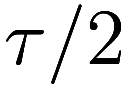to stay on the horizontal axis, but have no concept of other angles. Alternatively, consider that they lack the holographic principle (ie, Cauchy’s Theorem) that shows that the values an integrable function takes in the interior of a region is fully determined by its values on the region’s boundary; that would be like constraining a real-integrable function given only two values at the ends of a line segment, which is impossible unless you restrict to linear functions. By analogy, imagine trying to hold a string rigid by holding its ends versus trying to hold a piece of paper rigid by holding its boundary.

## Order

The reals are ordered and can be partitioned into three subsets, called positive, negative, and the number zero, also known as “signs”. Here positive means to the right of zero in the standard orientation of the plane. It may be strange to be interested in a seemingly random geometric property but it turns out to be quite important in the reals.

Certainly the positive numbers and negative numbers are each closed under addition; but when considering the reals we further get relationships between multiplication and a number’s sign, eg any negative real times a positive real is a negative real.

Using these signs we can define an order, wherein we write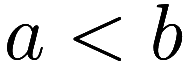for reals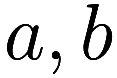to mean that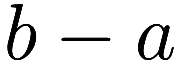is positive. This relationship is transitive, so thatand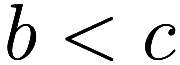implies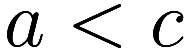5because the sum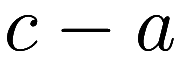of two positive numbersand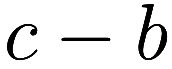is positive. True fact: I received negative infinity points on an informal math exam in 2005 for failing to make this observation.. This apparently banal observation is an important foundation to understanding real numbers, as will be discussed very shortly.

## Algebra

It is only natural to ask then what further wonders results if one were to again split reals into two parts, and so on.

Despite many inventive attempts to “halve” the reals, the remarkable Artin-Schreier Theorem (see also real closed fields) shows that it is impossible! Let us take a closer look.

We of course remember the Fundamental Theorem of Algebra that all polynomials have a root. This theorem no longer holds in the reals; consider, for example,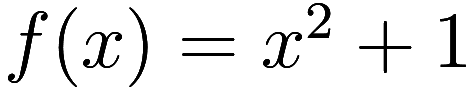. We know the two roots of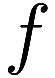are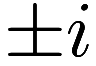, but neither of those are real numbers, and if the reals had some other root forthenwould have at least three roots, impossible as it is degree 2.

In fact this gives us a much cleaner way to interpret the sign of a real: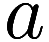is positive if and only if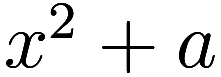has no real roots.

Should we despair of ever finding roots of real polynomials? No, for every polynomial of odd degree with real coefficients has a real root. This odd fact is actually an easy consequence of the order properties of the real numbers.

Now the Artin-Schreier Theorem shows that this situation is essentially unique to the reals, or objects formally isomorphic to the reals, and so one can go no “deeper”. Formally, if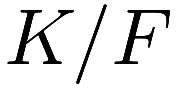is a nontrivial finite degree field extension and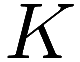is algebraically closed (ie it satisfies the FTA), then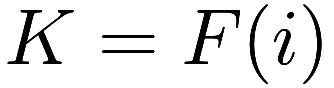and so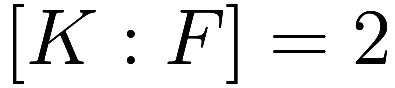. This implies that the field of real numbers is not a finite degree extension of any proper field.

## To infinity… and beyond

We are familiar with having a single “point at infinity” giving the Riemann sphere, but when doing analysis on the reals it can be more natural to have two different infinities, one positive and one negative! And if two is not enough, further madness can be sought in the long real line or the field of hyperreals.

Hopefully this article gives an accessible first taste of the real numbers, showing both the ways in which they are familiar, and also a glimpse of some of their exotic properties which have yielded much exciting mathematics. There is much I have omitted, from graphing functions by drawing a path in the plane, to constructing real numbers using Dedekind cuts, integration using Riemann sums instead of contours, the existence of finitely-supported smooth functions, and much more.

Update: Thanks /u/almightySapling for identifying an error